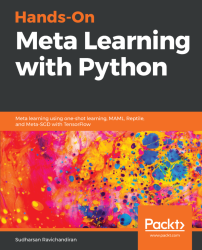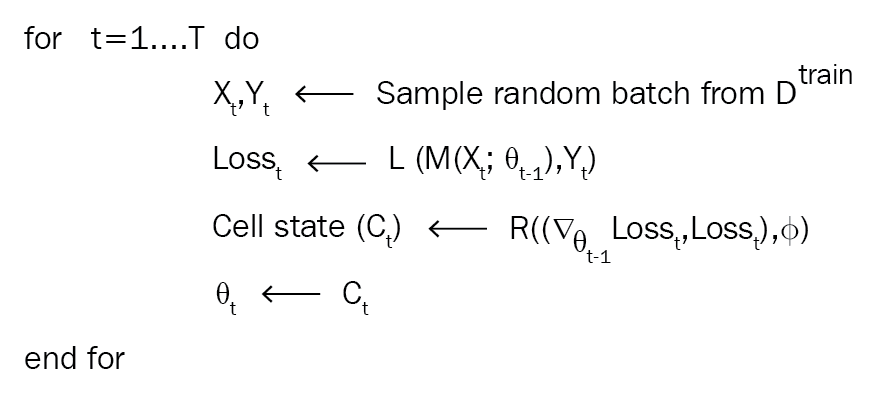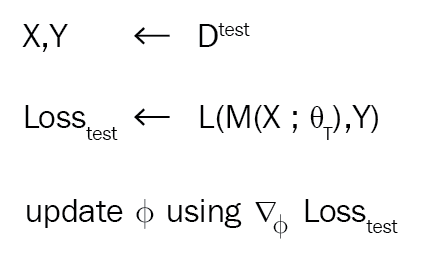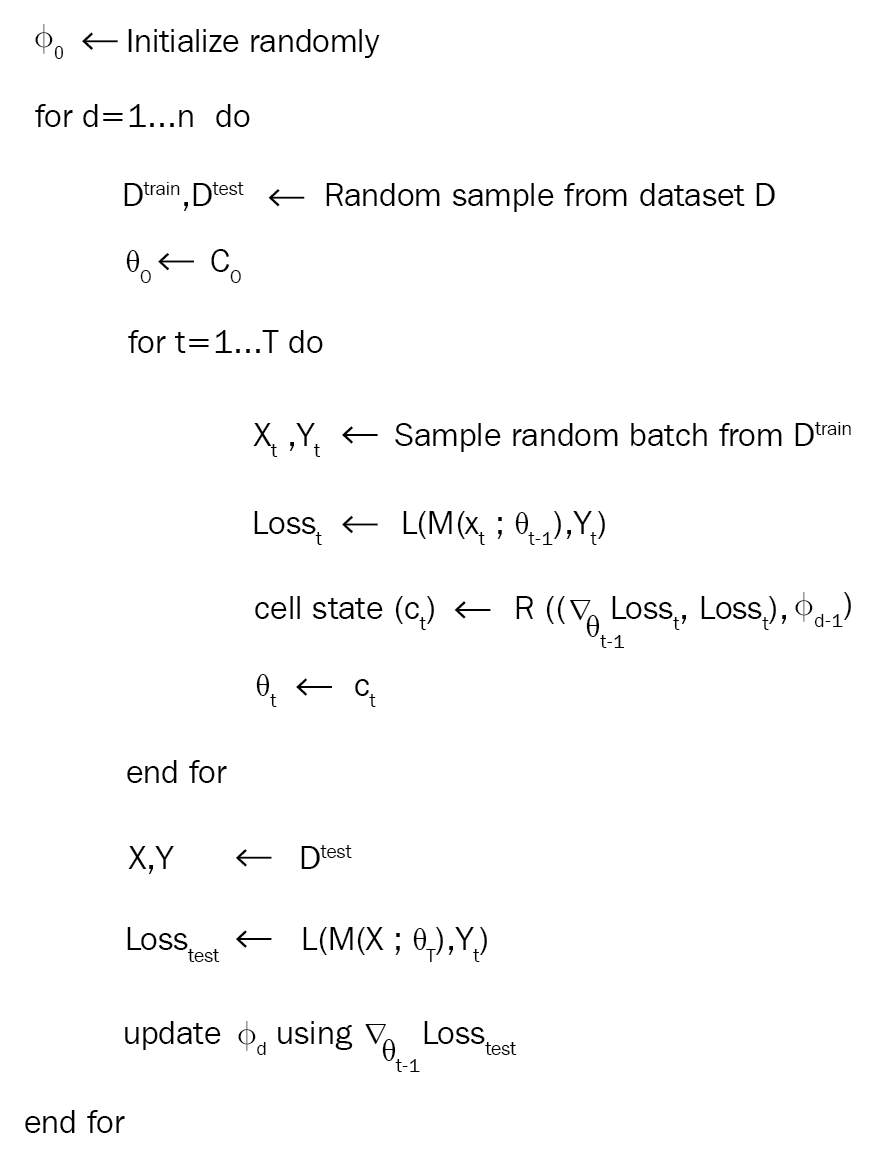•#### Hands-On Meta Learning with Python#### Overview of this book

Meta learning is an exciting research trend in machine learning, which enables a model to understand the learning process. Unlike other ML paradigms, with meta learning you can learn from small datasets faster. Hands-On Meta Learning with Python starts by explaining the fundamentals of meta learning and helps you understand the concept of learning to learn. You will delve into various one-shot learning algorithms, like siamese, prototypical, relation and memory-augmented networks by implementing them in TensorFlow and Keras. As you make your way through the book, you will dive into state-of-the-art meta learning algorithms such as MAML, Reptile, and CAML. You will then explore how to learn quickly with Meta-SGD and discover how you can perform unsupervised learning using meta learning with CACTUs. In the concluding chapters, you will work through recent trends in meta learning such as adversarial meta learning, task agnostic meta learning, and meta imitation learning. By the end of this book, you will be familiar with state-of-the-art meta learning algorithms and able to enable human-like cognition for your machine learning models.
Title PageDedicationContributorsPrefaceFree Chapter
Introduction to Meta LearningFace and Audio Recognition Using Siamese NetworksPrototypical Networks and Their VariantsRelation and Matching Networks Using TensorFlowMemory-Augmented Neural NetworksMAML and Its VariantsMeta-SGD and ReptileGradient Agreement as an Optimization ObjectiveAssessmentsOther Books You May EnjoyIndex## Optimization as a model for few-shot learning

We know that, in few-shot learning, we learn from lesser data points, but how can we apply gradient descent in a few-shot learning setting? In a few-shot learning setting, gradient descent fails abruptly due to very few data points. Gradient descent optimization requires more data points to reach the convergence and minimize loss. So, we need a better optimization technique in the few-shot regime. Let's say we have amodel parameterized by some parameter. We initialize this parameterwith some random values and try to find the optimal value using gradient descent. Let's recall the update equation of our gradient descent:In the previous equation, the following applies:

•is the updated parameter
•is the parameter value at previous time step
•is the learning rate
•is the gradient of loss function with respect toDoesn't the update equation of gradient descent look familiar? Yes, you guessed it right: it resembles the cell state update equation of LSTM and it can be written as follows:We can totally relate our LSTM cell update equation with gradient descent as, let's say= 1, then the following applies:So, instead of using gradient descent as an optimizer in the few-shot learning regime, we can use LSTM as an optimizer. Our meta learner is the LSTM, which learns the update rule for training our model. So we use two networks: one, our base learner, which learns to perform a task, and the other, the meta learner, which tries to find the optimal parameter. But how does this work?

We know that, in LSTM, we use a forget gate for discarding information that is not required in the memory, and it can be represented as follows:How can this forget gate be useful in our optimization setting? Let's say we are in a position where the loss is high, and the gradient is close to zero. How can we escape from this position? In this case, we can shrink the parameters of our model and forget some parts of its previous value. So, we can use our forget gate to do that and it takes a current parameter value, current lossand the previous forget gate as the input; it can be represented as follows:Now let's come to the input gate. We know that the input gate in LSTM is used for deciding what value to update, and it can be represented as follows:In our few-shot learning setting, we can use this input gate to tune our learning rate to learn quickly while preventing it from divergence:So, our meta learner learns the optimal value ofandBut still, how does this work?

Let's say we have a base networkparameterized byand our LSTM meta learnerparameterized by. Assume that we have a dataset. We split our dataset asandfor training and testing respectively. First, we randomly initialize our meta learner parameter.

For some T number of iterations, we randomly sample data points from, calculate the loss, and then we calculate the gradients of loss with respect to our model parameter. Now we feed this gradient, loss, and meta learner parameterto our meta learner. Our meta learnerwill return a cell stateand then we update our base networkparameterat a time t as.We repeat this for some N number of times, as shown in the following diagram:So, after T iterations, we will have an optimal parameter. But how can we check the performance ofand how can we update our meta learner parameter? We take the test set and compute the loss on our test set with parameter. Then, we calculate the gradients of the loss with respect to our meta learner parameterand then we update, as shown here:We do this for some n number of iterations and update our meta learner. The overall algorithm is shown here: